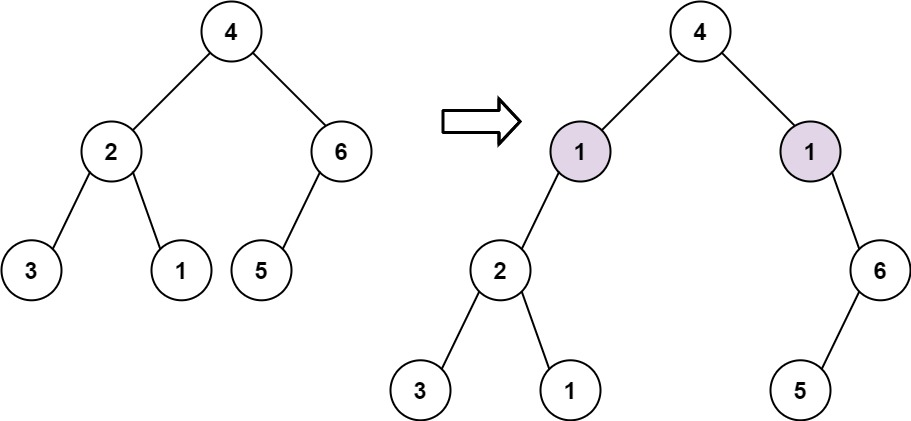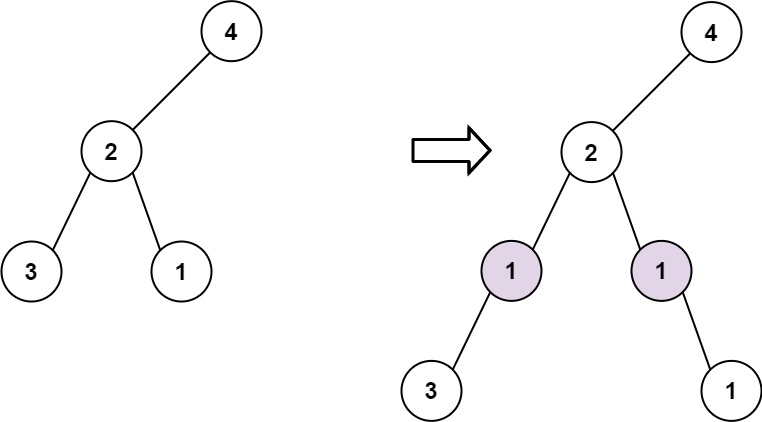# 623. Add One Row to Tree

Medium
Given the `root` of a binary tree and two integers `val` and `depth`, add a row of nodes with value `val` at the given depth `depth`.
Note that the `root` node is at depth `1`.
• Given the integer `depth`, for each not null tree node `cur` at the depth `depth - 1`, create two tree nodes with value `val` as `cur`'s left subtree root and right subtree root.
• `cur`'s original left subtree should be the left subtree of the new left subtree root.
• `cur`'s original right subtree should be the right subtree of the new right subtree root.
• If `depth == 1` that means there is no depth `depth - 1` at all, then create a tree node with value `val` as the new root of the whole original tree, and the original tree is the new root's left subtree.
Example 1:Input: root = [4,2,6,3,1,5], val = 1, depth = 2
Output: [4,1,1,2,null,null,6,3,1,5]
Example 2:Input: root = [4,2,null,3,1], val = 1, depth = 3
Output: [4,2,null,1,1,3,null,null,1]
Constraints:
• The number of nodes in the tree is in the range `[1, 10^4]`.
• The depth of the tree is in the range `[1, 10^4]`.
• `-100 <= Node.val <= 100`
• `-10^5 <= val <= 10^5`
• `1 <= depth <= the depth of tree + 1`

### 解題

Runtime: 2 ms, faster than 100%
Memory Usage: 5.6 MB, less than 50%
/**
* Definition for a binary tree node.
* type TreeNode struct {
* Val int
* Left *TreeNode
* Right *TreeNode
* }
*/
func addOneRow(root *TreeNode, val int, depth int) *TreeNode {
if root == nil { return nil }
if depth == 1 {
return &TreeNode{ Val: val, Left: root}
}
if depth == 2 {
newLeft := &TreeNode{ Val: val, Left: root.Left }
newRight := &TreeNode{ Val: val, Right: root.Right }
return &TreeNode{ Val: root.Val, Left: newLeft, Right: newRight }
}